# Circuit Equations:Transformer

The application of the voltage law to both primary and secondary circuits of a transformer gives: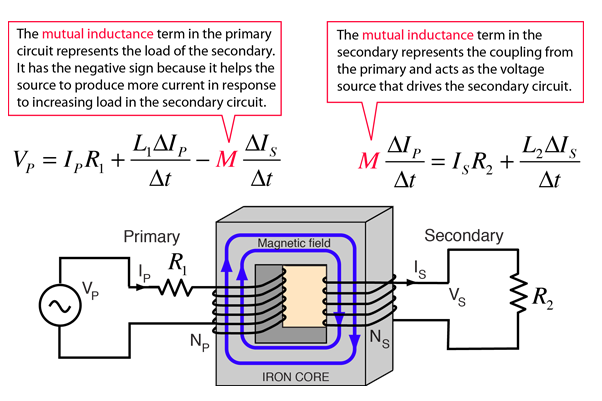The transformer is the most common application of the concept of mutual inductance. In the transformer, the effect of the mutual inductance is to cause the primary ciruit to take more power from the electrical supply in response to an increased load on the secondary. For example, if the load resistance in the secondary is reduced, then the power required will increase, forcing the primary side of the transformer to draw more current to supply the additional need.

 Circuit Equation Solutions Calculation
Index

Transformer concepts

Inductance concepts

 HyperPhysics***** Electricity and Magnetism R Nave
Go Back

# Circuit Equations: Transformer

The secondary circuit equation is that of an RL circuit driven by a voltage source: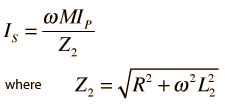The primary circuit current is given by:ShowNote that the effective impedance of the primary circuit contains not only the coupling of the mutual impedance, but contains terms dependent upon the secondary load resistor R. When R is smaller (greater load) , ZP becomes smaller and more resistive, taking more power from the primary source.

For ideal coupling, the mutual inductance becomes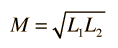Development of Equations Numerical Example Power Calculation
Index

Transformer concepts

Inductance concepts

AC Circuits

 HyperPhysics***** Electricity and Magnetism R Nave
Go BackRSOhms VPVolts VSVolts IPAmps ISAmps PPWatts PSWatts Open 119 238 3.2 0 50 0 100 99 198 4.8 2.0 114 395 20 60 120 12.1 6.0 729 717 5 24 48 19.2 9.6 1844 460 1 5.7 11.4 22.9 11.4 2612 131

 Development of Equations Plot of Data Calculation
Index

Transformer concepts

Inductance concepts

AC Circuits

 HyperPhysics***** Electricity and Magnetism R Nave
Go BackIndex

Transformer concepts

Inductance concepts

AC Circuits

 HyperPhysics***** Electricity and Magnetism R Nave
Go Back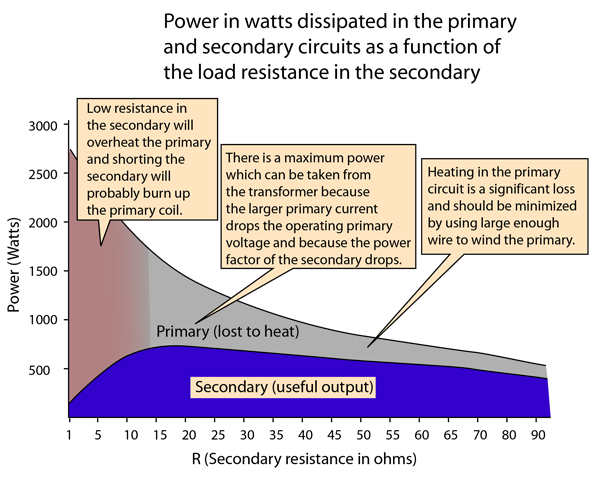There is a practical midrange of load resistances for the operation of a transformer. In the example above, you can see that maximum output power is obtained with a load resistance between 15 and 20 ohms. When too heavy a load is drawn from the secondary, the primary coil heats up and the amount of audible "hum" that you hear from the transformer increases. My brother, Edgar Nave, worked in commercial electrical construction and wired many large transformers. He reports incidents when the secondary of a transformer was shorted, and says that the transformer would "squawl" like a wounded beast before it burned up the primary coil.

 Data table and explanation Plot and circuit diagram Calculation
Index

Transformer concepts

Inductance concepts

AC Circuits

 HyperPhysics***** Electricity and Magnetism R Nave
Go Back

# Circuit Equations:Transformer

The application of the voltage law to both primary and secondary circuits of a transformer gives:Obtaining the circuit equations requires the simultaneous solution of the primary and secondary circuit equations. That solution is facilitated by using the complex impedance method, presuming a sinusoidal supply voltage with angular frequency ω. Standard AC practice uses rms currents and voltages, and in the complex impedance approach the derivative of current dI/dt = jωI. The primary and secondary loop equations then take the form:From the secondary circuit equation we can find an expression for the secondary current IS.This can be substituted into the primary loop equation:The last term can be rationalized by multiplying numerator and denominator by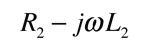givingThis allows us to separate the real and imaginary parts and place the expression in the form of a standard complex number.This can be written in the form of a standard AC circuit impedance Z:whereTransformer Circuit Equations Circuit Equation Solutions Calculation
Index

Transformer concepts